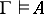# Logical consequence

(diff) ← Older revision | Latest revision (diff) | Newer revision → (diff)
A proposition that is true for any interpretation of the non-logical symbols (that is, the names (cf. Name) of objects, functions, predicates) for which the premises are true. If a propositionis a logical consequence of a set of propositions, one says thatlogically implies, or thatfollows logically from.
Ifis a set of statements of a formalized first-order logico-mathematical language (cf. Logico-mathematical calculus) andis a proposition of this language, then the relation "A is a logical consequence of G" means that any model foris a model for. This relation is denoted by. The Gödel completeness theorem of classical predicate calculus implies that the relationcoincides with the relation, that is,if and only ifis deducible fromby the methods of classical predicate calculus.